Latest Banking jobs   »   Quantitative Aptitude Quiz For IBPS RRB...

# Quantitative Aptitude Quiz For IBPS RRB PO, Clerk Prelims 2021- 1st May

Q1. ‘A’ invested Rs. 5,000 more than that of ‘B’, while ratio of investment period for ‘A’ and ‘B’ is 2 : 1. If out of total profit of Rs. 2450, ‘A’ got Rs. 1750 then find total investment done by ‘A’ and ‘B’ together
(a) Rs. 20,000
(b) Rs. 45,000
(c) Rs. 25,000
(d) Rs. 30,000
(e) Rs. 40,000

Q2. Neeraj’s income is 30% more than Veer’s income which is Rs.6000 more than Satish’s income. If ratio between Neeraj’s income to Satish’s income is 39 : 20, then find Veer’s income
(a) Rs. 9,000
(b) Rs. 12,000
(c) Rs. 18,000
(d) Rs. 15,000
(e) Rs. 21,000

Q3. A and B invest in the ratio of 3 : 5. After 6 months, C joins the business by investing some amount. At the end of the year, the profit share of B and C are equal. Find initial investment of A is what percent of the initial investment of C.
(a) 24%
(b) 36%
(c) 60%
(d) 45%
(e) 30%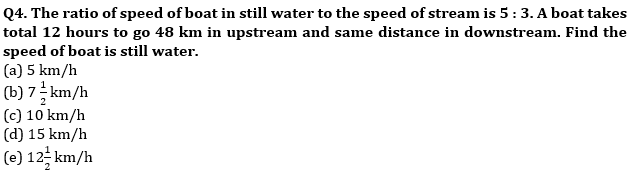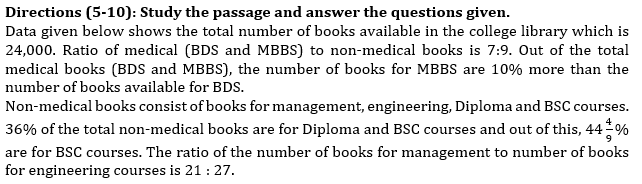Q5. The number of books available for engineering course is how much more or less than the number of books available for BDS course
(a) 120
(b) 140
(c) 160
(d) 170
(e) 180

Q6. Find the ratio of the total number of books available for MBBS and Diploma courses together to the total number of books available for management and engineering together?
(a) 205 : 216
(b) 216 : 205
(c) 26 : 27
(d) 23 : 24
(e) 209 : 216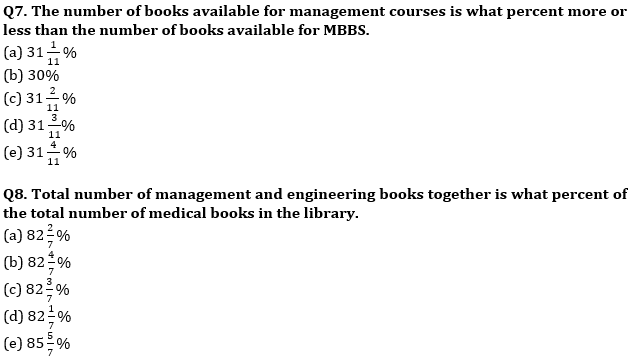Q9. Find the difference between the total number of books for BDS and management courses together and the total number of books for engineering, BSC and Diploma courses together?
(a) 960
(b) 950
(c) 940
(d) 980
(e) 930

Q10. Total number of engineering books are further classified as books for M. Tech courses and books for B. Tech courses which are in ratio 5 : 7 (M. Tech : B. Tech). Number of books for B.Tech is what percent of the number of books for BDS?
(a) 55.7%
(b) 56.7%
(c) 50.6%
(d) 62.6%
(e) 57.6%

Q11. Veer and Bhavya started a business by investing Rs 45,000 and Rs 50,000 respectively. At the end of the year, they decided to divide 50% of the total profit share equally and rest in the investment ratio. If they had divided entire profit share in investment ratio, Bhavya got Rs 1500 more profit than that of actual, then find the total profit share?
(a) Rs 1,04,000
(b) Rs 1,08,000
(c) Rs 1,12,000
(d) Rs 1,14000
(e) Rs 1,15000

Q12. The average weight of boys in a class of total strength 50 is 40 kg while average weight of girls is 35 kg. Find number of girls in class if average weight of class is 38 kg.
(a) 10
(b) 30
(c) 20
(d) 40
(e) 15

Q13. ‘A’ has a certain average for 9 innings. In the tenth innings he scores 100 runs thereby increasing his average by 8 runs. His new average is
(a) 20
(b) 24
(c) 28
(d) 32
(e) None of these

Q14. Veer , Sameer & Gopal started a business with initial investment in the ratio of 10 : 12 : 9 respectively. At the end of one year Veer , Sameer & Gopal withdrew Rs. 1000, Rs. 1200 and Rs. 1500 respectively from their initial investment. If at the end of two years Sameer got Rs. 16200 as profit share out of total profit of Rs. 40950, then find the initial investment of Gopal?
(a) 4500 Rs.
(b) 4200 Rs.
(c) 3600 Rs.
(d) 3200 Rs.
(e) 4800 Rs.

Q15. Three friends Abhi, Archit & Nik enter into a business. Abhi contributes 1/3rd of total investment and Archit contributes as much as Abhi & Nik contributes together. Total profit at end of year is given as Rs 6600. Find share of profit of Nik?
(a) Rs 550
(b) Rs 1100
(c) Rs 900
(d) Rs 3300
(e) Rs 2200

Q16. The average age of 16 student in a class is x year. If a teacher whose age is 54 years joined the class than average of the class increases by 2 years. Find the value of x?
(a) 18 years
(b)25 year
(c) None of these
(d) 20 years
(e) 30 years

Q17. Ratio of upstream speed to downstream speed is 1 : 11. If speed of boat in still water is 30 km/hr then find the distance covered in upstream in 5 hours ? (in km)
(a) 66
(b) 55
(c) 25
(d) 30
(e) 40

Q18. The speed of boat in still water is 700% more than speed of stream. Boat travels distance of 63 km in downstream in 2 hr 48 min. Then, find the time taken by boat to travel 56 km in upstream.
(a) 2 hr 56 min
(b) 3 hr 02 min
(c) 3 hr 12 min
(d) 3 hr 36 min
(e) 4 hr 12 min

Q19. A boatman can cover a river of 60 km length and came back at its initial point in 4.5 hrs. If speed of boat is thrice than that of the speed of stream then find the speed of boat?
(a) 10
(b) 30
(c) 20
(d) 60
(e) 25

Q20. If the price of milk is increased by 25% then a person can buy 8 litres less milk by spending Rs 160. Find the final rate of milk?
(a) Rs 4 per litre
(b) Rs 5 per litre
(c) Rs 8 per litre
(d) Rs 6 per litre
(e) Rs 7 per litre

Practice More Questions of Quantitative Aptitude for Competitive Exams:

###### Study Plan for IBPS RRB PO/Clerk Prelims 2021

Solutions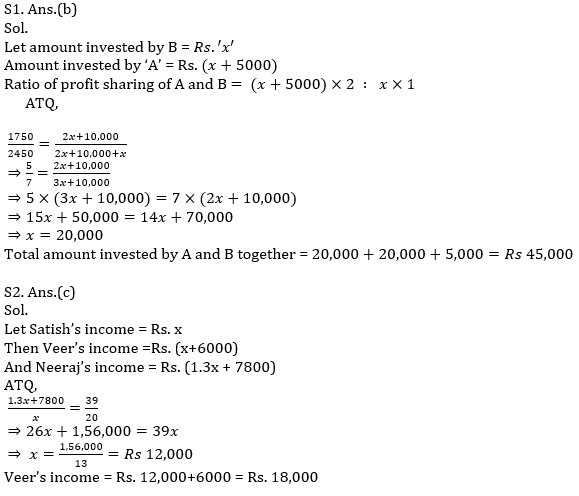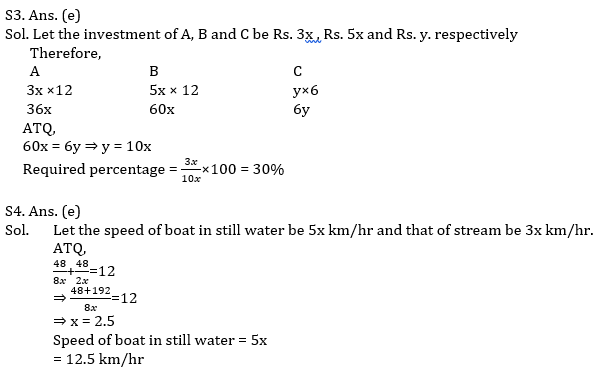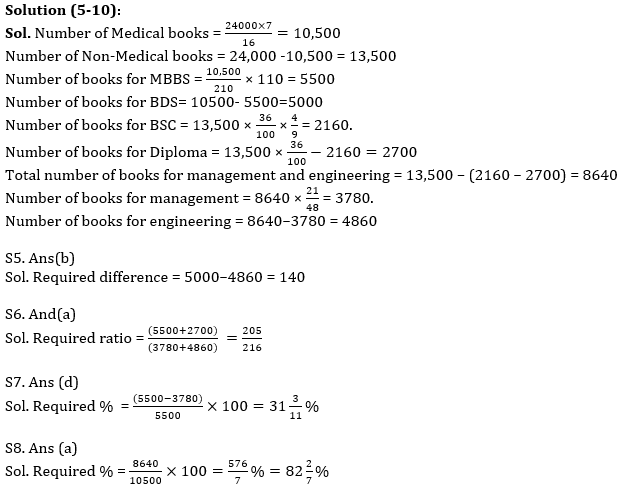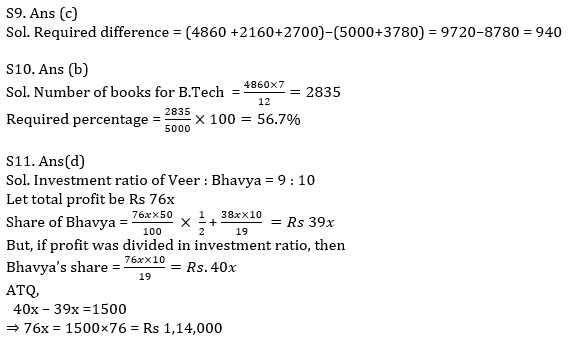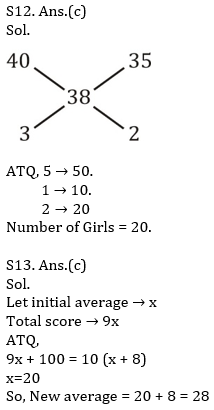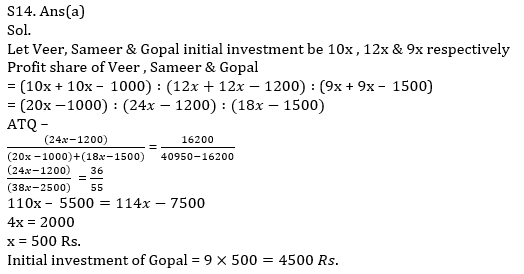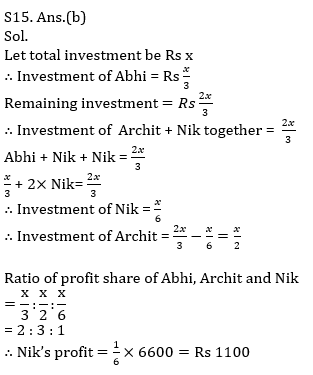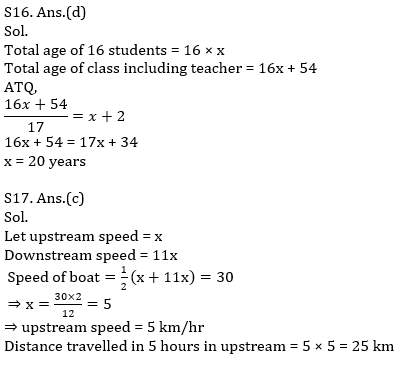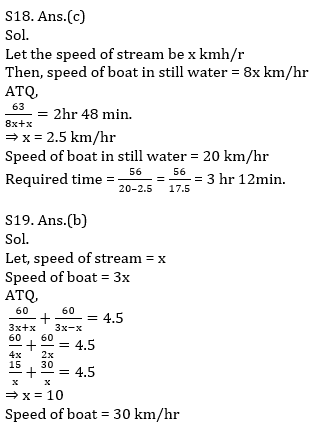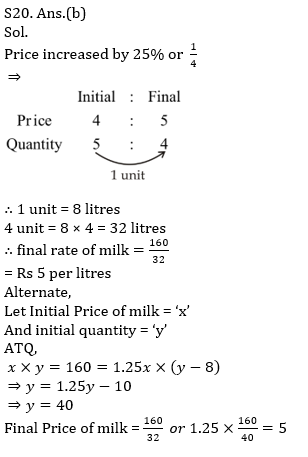#### Congratulations!Incorrect details? Fill the form again here

•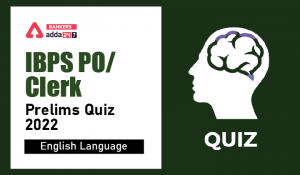English Quizzes For IBPS Clerk/PO Prelim...
•English Quizzes For IBPS Clerk/PO Prelim...
•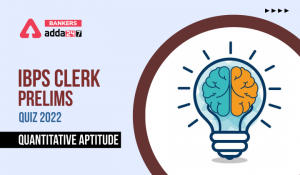Quantitative Aptitude Quiz For IBPS Cler...
•Quantitative Aptitude Quiz For IBPS Cler...
•Reasoning Ability Quiz For IBPS Clerk/PO...
•Quantitative Aptitude Quiz For IBPS Cler...